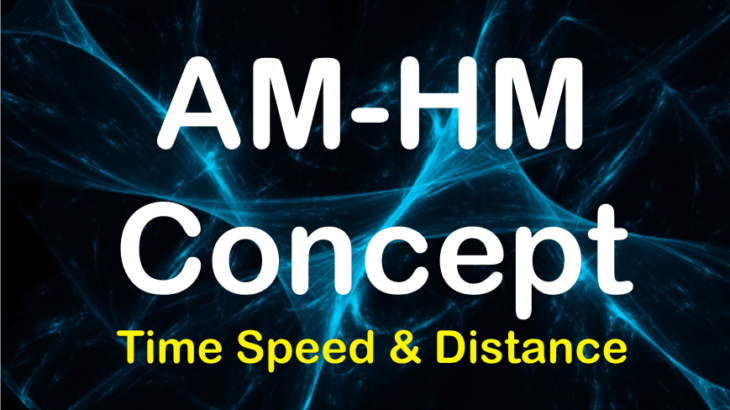# AM-HM Concept : Time Speed and Distance

CAT 2020 MOCK Test Series at INR 1000/- only.

## AM-HM Concept:

The AM-HM concept states that if the speeds are in arithmetic progression/ harmonic progression then, the corresponding time taken are in harmonic progression /arithmetic progression and vice versa.

Note: We use this AM-HM concept only when the same distance is traveled at different speeds.

Here is the proof of AM-HM Concept
Say, a distance ‘d’ is covered by A, B and C with speeds S1, S2 and S3 respectively, and the respective time taken are T1, T2. and T3. We have the following relations

${S_1} = \frac{d}{{{T_1}}}$, or ${S_1} \propto \frac{1}{{{T_1}}}$

${S_2} = \frac{d}{{{T_2}}}$, or ${S_2} \propto \frac{1}{{{T_2}}}$

${S_3} = \frac{d}{{{T_3}}}$, or ${S_3} \propto \frac{1}{{{T_3}}}$

Observe that if, S1, S2, and S3 are in Arithmetic Progression then, the corresponding times T1, T2, and T3 are in Harmonic Progression and vice-versa.

### Let us take a few examples to see the application.

Example 1: Arun, Barun, and Kiranmala start from the same place and travel in the same direction at speeds of 30, 40 and 60 km per hour respectively. Barun starts two hours after Arun. If Barun and Kiranmala overtake Arun at the same instant, how many hours after Arun did Kiranmala start?

Solution:

Note that all three travel the same distance when Kiranmala overtakes both Arun and Barun. Also, their speeds, i.e. 30, 40, and 60 are in harmonic progression. Therefore, according to AM-HM concept, the time taken by them will be in arithmetic progression.

So, if Barun starts 2 hours after Arun, the common difference between the time taken by Arun and Barun is also 2 hours. Therefore, the time taken by Kiranmala should also have the same difference to keep the time taken by all in arithmetic progression. Hence, Kiranmala starts 2 hours after Barun or 4 hours after Arun.

Example 2: If a man cycles at 10 km/hr, then he arrives at a certain place at 1 p.m. If he cycles at 15 km/ hr, he will arrive at the same place at 11 a.m. At what speed must he cycle to get there at noon?

Solution:

Observe that the time taken by the man in all three scenarios is in arithmetic progression with common difference of 1 hour. Therefore, according to AM-HM concept, the corresponding speeds will be in Harmonic progression.

Or Speed needed to travel to reach at 12 pm = Harmonic mean of 10 km/hr and 15 km/hr. i.e. $\frac{{2 \times 10 \times 15}}{{10 + 15}}$= 12km/hr.

Example 3: On an escalator which is moving in an upward direction, Ramlal takes 20 seconds to reach at the top. He again walks on the same upward moving escalator to reach at the bottom, and this time he takes 60 seconds. If the escalator is stopped, how much time he will take to walk up the escalator.

(Assume that his walking speed is same in both upward and downward direction)

Solution:

The distance traveled by the speeds (x+y) and (x-y) is the same. And we have to find the time taken to cover this distance with the speed x.

Note that the terms (x+y), x, and (x-y) are in Arithmetic progression. Accordingly, the respective time taken will be in Harmonic progression.

Therefore, applying the AM-HM concept, the time taken with speed x = Harmonic mean of time taken with speeds (x+y) and (x-y) i.e.

Time taken = $\frac{{2 \times 20 \times 60}}{{20 + 60}}$=30 seconds.